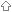Last update July 10, 2004

# Dwith Swig / ProblemTable of contents of this pageProblemSolutionImplementation

###ProblemThe items in DwithSwig/Modifications are not sufficient. There are some things which work when two classes are in the same file, but not if they are in separate files, as in the case of these generated by SWIG. The problem area concerns a static member function which is put into each class by SWIG. In the automatically generated code there is a private static function which returns a pointer held within the object:``` public class A { private void* Ptr; private static void* getPtr(A obj) { return (obj === null) ? null : Ptr; } }```

If a second class B has a constructor with an A as an argument the code generated automatically is``` public class B { // ... public this(A a) { // contains A.getPtr(a) which fails unless the code for B is in the same file as the code for A. // This applies even if I change the permission of the static function to public. } }```

The problem is that the import A and the class A have the same name.

###SolutionThe solution is to modify the automatically generated code in two ways. First, make the static function public``` public class A { private void* Ptr; public static void* getPtr(A obj) { return (obj === null) ? null : Ptr; } }```

Second, modify the reference in class B from A.getPtr(a) to A.A.getPtr(a) where the first A represents the import name and the second A is the class name. I have learned something about D.

As before, I would like the code to be generated automatically. I have to change the part of SWIG which generates the D code so that it does the correct thing.

###ImplementationI have implemented this by changing the name of the file generated for each class. I have added the work class before the name so that the files become e.g. classA.d. One reason for this is that it means that there is now no difference between a reference in the class itself or in another class. The interface file now needs commands such as the following to generate the import instructions.`%pragma(dmd) classimports="import classA;"`

| | | | | | | | | |

(date of last change: July 10, 2004 17:25 )